## 假说表示

Python代码实现

``````import numpy as np

def sigmoid(z):
return 1 / (1 + np.exp(-z))
``````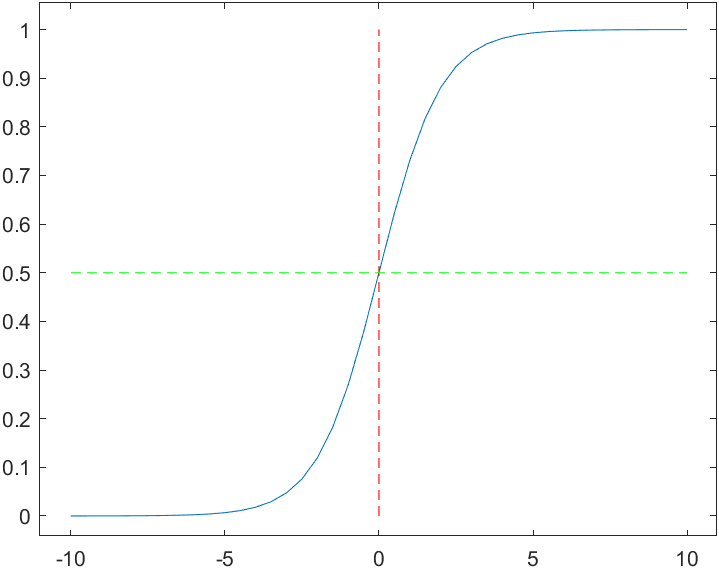`h_theta(x)`的作用是，对于给定的输入变量，根据选择的参数计算输出变量=1的可能性（estimated probablity）即`h_theta(x) = P(y=1 | x;theta)`

## 判定边界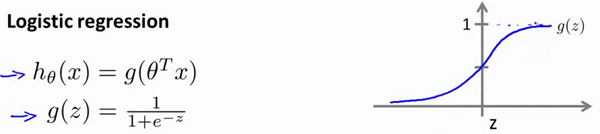• `h(x) >= 0.5`时，预测`y = 1`
• `h(x) < 0.5`时，预测`y = 0`

• `z = 0``g(z) = 0.5`
• `z > 0``g(z) > 0.5`
• `z < 0``g(z) < 0.5`

`z = theta'x`，即：`theta'x >= 0`时，预测`y = 1``theta'x < 0`时，预测`y =0`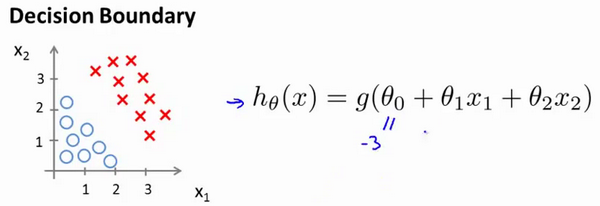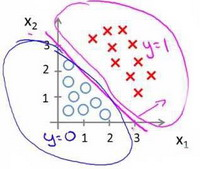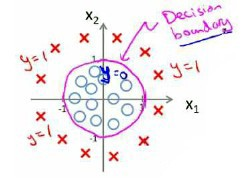`h_theta(x) = g(theta0 + theta1x1 + theta2x2 + theta3x1^2 + theta4x2^2)`

## 代价函数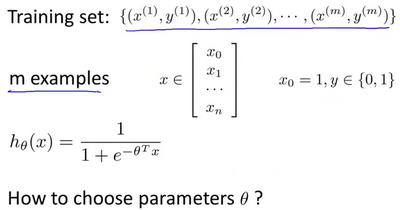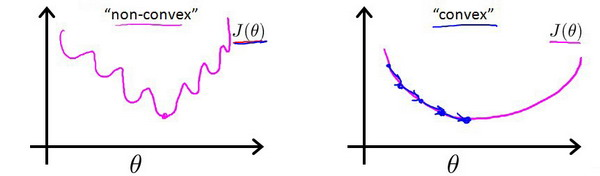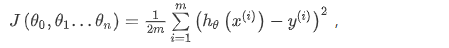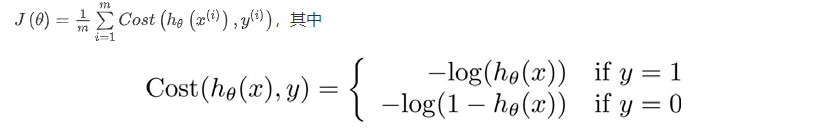`h(x)``Cost(h(x), y)`之间的关系如图所示• 当实际的`y=1``h(x)`也为 1 时误差为 0
• `y=1``h(x)`不为1时误差随着`h(x)`变小而变大；
• 当实际的`y=0``h(x)`也为 0 时代价为 0
• `y=0``h(x)`不为 0 时误差随着`h(x)`的变大而变大。

`Cost(h(x),y) = -y*log(h(x)) - (1-y)*log(1-h(x))`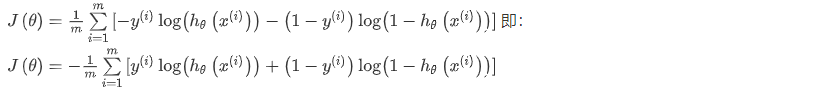Python实现

``````import numpy as np

def cost(theta, X, y):

theta = np.matrix(theta)
X = np.matrix(X)
y = np.matrix(y)
first = np.multiply(-y, np.log(sigmoid(X* theta.T)))
second = np.multiply((1 - y), np.log(1 - sigmoid(X* theta.T)))
return np.sum(first - second) / (len(X))
``````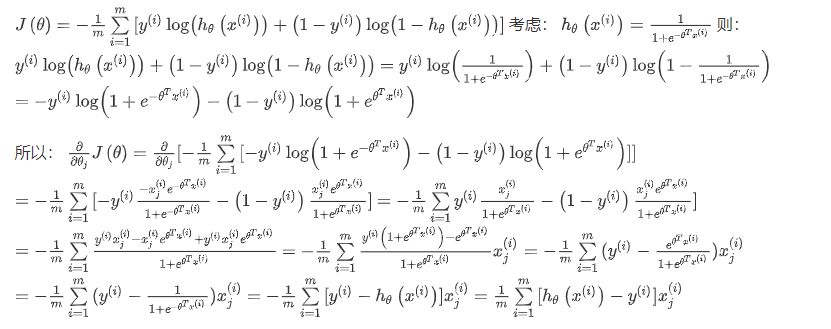``````function [jVal, gradient] = costFunction(theta)
​
jVal = [...code to compute J(theta)...];
gradient = [...code to compute derivative of J(theta)...];

end

options = optimset('GradObj', 'on', 'MaxIter', '100');

initialTheta = zeros(2,1);

[optTheta, functionVal, exitFlag] = fminunc(@costFunction, initialTheta, options);
``````

## 简化的成本函数和梯度下降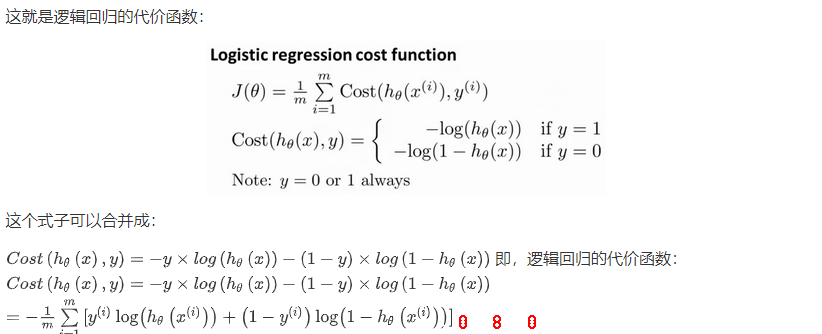## 高级优化``````function [jVal, gradient]=costFunction(theta)

jVal=(theta(1)-5)^2+(theta(2)-5)^2;

end
``````

``````options=optimset('GradObj','on','MaxIter',100);
​
initialTheta=zeros(2,1);

[optTheta, functionVal, exitFlag]=fminunc(@costFunction, initialTheta, options);
``````

## 多类别分类：一对多